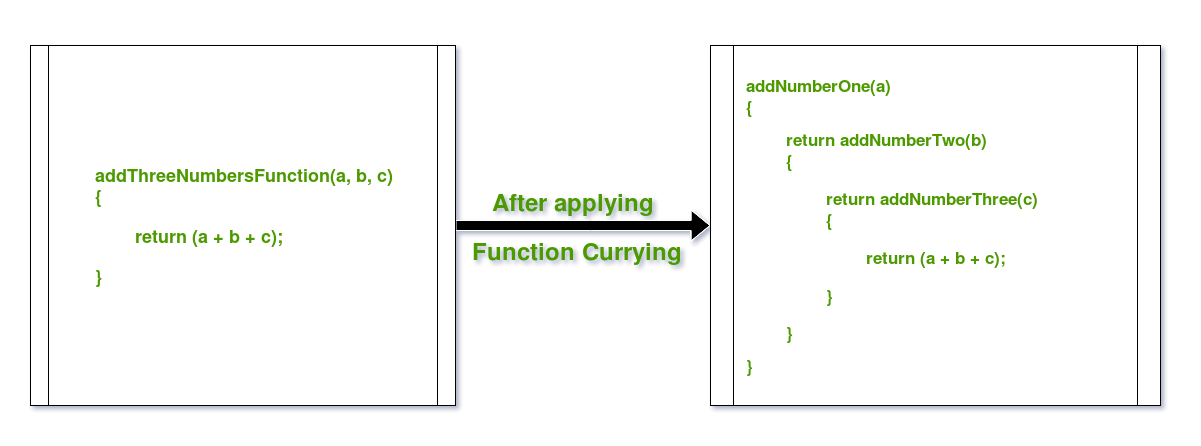GeeksforGeeks App
Open AppBrowser
Continue

# Currying Functions in Java with Examples

Function Currying is a concept of breaking a function with many arguments into many functions with single argument in such a way, that the output is same. In other words, its a technique of simplifying a multi-valued argument function into single-valued argument multi-functions.

Consider the example to clear the concept:Currying breaks down higher order functions into a series of smaller cascaded functions which take in one argument and return a function except for the last cascaded function which returns the desired value.

For example:

Let there be a function which maps asCurrying the above function will produceThusmaps fromto a function which in turn maps fromtoThe above mathematical expression can also be represented as:Hence,Below are some examples in Java to demonstrate Function Currying:

Example 1: Adding 2 numbers using Function Currying

 `// Java Program to demonstrate Function Currying`` ` `import` `java.util.function.Function;`` ` `public` `class` `GFG {``    ``public` `static` `void` `main(String args[])``    ``{`` ` `        ``// Using Java 8 Functions``        ``// to create lambda expressions for functions``        ``// and with this, applying Function Currying`` ` `        ``// Curried Function for Adding u & v``        ``Function >``            ``curryAdder = u -> v -> u + v;`` ` `        ``// Calling the curried functions`` ` `        ``// Calling Curried Function for Adding u & v``        ``System.out.println(``"Add 2, 3 :"``                           ``+ curryAdder``                                 ``.apply(``2``)``                                 ``.apply(``3``));`` ` `        ``}``}`

Output:

```Add 2, 3 :5
```

Example 2: Multiplying 2 numbers using Function Currying

 `// Java Program to demonstrate Function Currying`` ` `import` `java.util.function.Function;`` ` `public` `class` `GFG {``    ``public` `static` `void` `main(String args[])``    ``{`` ` `        ``// Using Java 8 Functions``        ``// to create lambda expressions for functions``        ``// and with this, applying Function Currying`` ` `        ``// Curried Function for Multiplying u & v``        ``Function >``            ``curryMulti = u -> v -> u * v;`` ` `        ``// Calling the curried functions``        ` `        ``// Calling Curried Function for Multiplying u & v``        ``System.out.println(``"Multiply 2, 3 :"``                           ``+ curryMulti``                                 ``.apply(``2``)``                                 ``.apply(``3``));``    ``}``}`

Output:

```Multiply 2, 3 :6
```

Example 3: Adding 3 numbers using Function Currying

 `// Java Program to demonstrate Function Currying`` ` `import` `java.util.function.Function;`` ` `public` `class` `GFG {``    ``public` `static` `void` `main(String args[])``    ``{`` ` `        ``// Using Java 8 Functions``        ``// to create lambda expressions for functions``        ``// and with this, applying Function Currying`` ` `        ``// Curried Function for Adding u, v & w``        ``Function > >``            ``triadder = u -> w -> v -> u + w + v;`` ` `        ``// Calling the curried functions`` ` `        ``// Calling Curried Function for Adding u, v & w``        ``System.out.println(``"Add 2, 3, 4 :"``                           ``+ triadder``                                 ``.apply(``2``)``                                 ``.apply(``3``)``                                 ``.apply(``4``));``    ``}``}`

Output:

```Add 2, 3, 4 :9
```

My Personal Notes arrow_drop_up
Related Tutorials